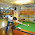### Semi-circle covering n points : Puzzle

Problem : (Posed by Saurabh Joshi, IIT Kanpur in his blog)

Given n points drawn randomly on the circumference of a circle, what is the probability they will all be within any common semicircle?

Solution :
Highlight the part between the * symbols for the answer.
*
If a semi-circle covering all n points, indeed exists, then, a semi-circle covering all n points and starting from one of the points in a clock-wise direction also exists.

So, given a semi-circle which starts at one of the point in clock-wise direction. The probability that the rest of the n-1 points will be in that semi-circle is 1/(2^(n-1)). So for n such semi-circle, the probability will be n/(2^(n-1)) *

:)

1.Understand that given that the semicircle starts at one of the points the probability is p=(0.5)^(n-1). Don't understand the n circle argument. It doesn't seem necessary that the semicircle starts from a point only. It could start somewhere between two points as well. Trying to understand the exact math here.

2.Actually i take that back. Semicircle cannot start from between two points.